## Improving the Beginner’s PID: Tuning Changes

(This is Modification #3 in a larger series on writing a solid PID algorithm)

### The Problem

The ability to change tuning parameters while the system is running is a must for any respectable PID algorithm.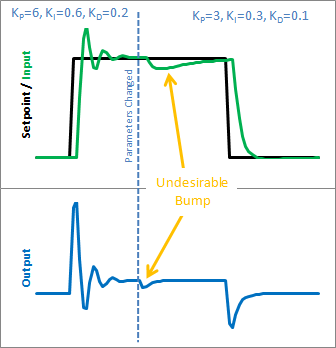The Beginner’s PID acts a little crazy if you try to change the tunings while it’s running. Let’s see why. Here is the state of the beginner’s PID before and after the parameter change above: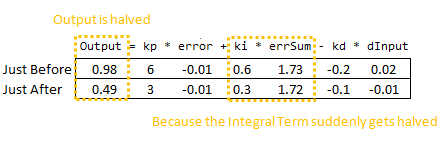So we can immediately blame this bump on the Integral Term (or “I Term”). It’s the only thing that changes drastically when the parameters change. Why did this happen? It has to do with the beginner’s interpretation of the Integral: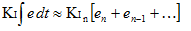This interpretation works fine until the Ki is changed. Then, all of a sudden, you multiply this new Ki times the entire error sum that you have accumulated. That’s not what we wanted! We only wanted to affect things moving forward!

### The Solution

There are a couple ways I know of to deal with this problem. The method I used in the last library was to rescale errSum. Ki doubled? Cut errSum in Half. That keeps the I Term from bumping, and it works. It’s kind of clunky though, and I’ve come up with something more elegant. (There’s no way I’m the first to have thought of this, but I did think of it on my own. That counts damnit!)

The solution requires a little basic algebra (or is it calculus?)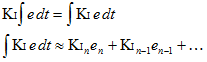Instead of having the Ki live outside the integral, we bring it inside. It looks like we haven’t done anything, but we’ll see that in practice this makes a big difference.

Now, we take the error and multiply it by whatever the Ki is at that time. We then store the sum of THAT. When the Ki changes, there’s no bump because all the old Ki’s are already “in the bank” so to speak. We get a smooth transfer with no additional math operations. It may make me a geek but I think that’s pretty sexy.

### The Code

```/*working variables*/
unsigned long lastTime;
double Input, Output, Setpoint;
double ITerm, lastInput;
double kp, ki, kd;
int SampleTime = 1000; //1 sec
void Compute()
{
unsigned long now = millis();
int timeChange = (now - lastTime);
if(timeChange>=SampleTime)
{
/*Compute all the working error variables*/
double error = Setpoint - Input;
ITerm += (ki * error);
double dInput = (Input - lastInput);

/*Compute PID Output*/
Output = kp * error + ITerm - kd * dInput;

/*Remember some variables for next time*/
lastInput = Input;
lastTime = now;
}
}

void SetTunings(double Kp, double Ki, double Kd)
{
double SampleTimeInSec = ((double)SampleTime)/1000;
kp = Kp;
ki = Ki * SampleTimeInSec;
kd = Kd / SampleTimeInSec;
}

void SetSampleTime(int NewSampleTime)
{
if (NewSampleTime > 0)
{
double ratio  = (double)NewSampleTime
/ (double)SampleTime;
ki *= ratio;
kd /= ratio;
SampleTime = (unsigned long)NewSampleTime;
}
}

```

So we replaced the errSum variable with a composite ITerm variable [Line 4]. It sums Ki*error, rather than just error [Line 15]. Also, because Ki is now buried in ITerm, it’s removed from the main PID calculation [Line 19].

### The Result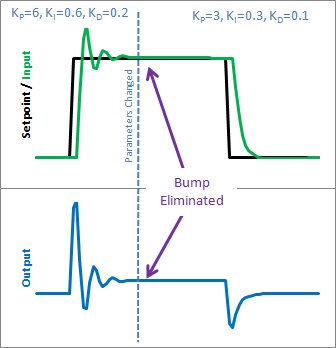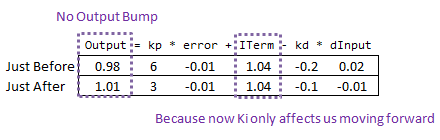So how does this fix things. Before when ki was changed, it rescaled the entire sum of the error; every error value we had seen. With this code, the previous error remains untouched, and the new ki only affects things moving forward, which is exactly what we want.
Next >>Tags: , ,

### 6 Responses to “Improving the Beginner’s PID: Tuning Changes”

1.Vasileios Kostelidis says:

I don’t get it. I am trying for about an hour but I cannot get it.
It looks the same arithmetically to me.

I don’t know what am I missing.

2.Brett says:

when you look at the calculus, it is identical. when it comes to implementation, it is different. let’s say that at t=10, Ki changes from 5 to 10, with Kp and Kd staying the same. now, let’s look at the integral term values at t=20:
old way: 10*(Integral of error from t=0 to 20)
new way: (Integral of (5*error) from t=0 to 10) + (Integral of (10*error) from t=10 to 20)

hopefully this helps reveal the difference. again. this is something you only notice if the value of Ki changes. if it stays the same, both methods are identical.

3.Vasileios Kostelidis says:

So, since:

old way: 10*(Integral of error from t=0 to 20)
new way: (Integral of (5*error) from t=0 to 10) + (Integral of (10*error) from t=10 to 20)

Then:

old way: 10*(Integral of error from t=0 to 20)
new way: 5*(Integral of error from t=0 to 10) + 10(Integral of error from t=10 to 20)

You are basically changing the algorithm. I kind of understand it now, but, is it OK to change the algorithm like that?

I know that this may very well be a silly question, but I don’t remember much from my control theory :).

Vasilis.

4.Brett says:

This entire series is about changing the algorithm. the idea is that the PID algorithm behaves in a predictable, reliable way, EXCEPT for in certain situations. The goal of these changes (all of them) is to make the algorithm work as you would expect, EVEN WHEN these special conditions occur.

5.Vasileios Kostelidis says:

OK, sounds nice. Thanks for the quick and accurate help!
Will let you know when my PID controller works.

6.Marcelo says:

The algebra is actually not correct. If both terms were equal, the result should be the same. Your ki is time dependent, which is why it cannot get out of the integrand. Still, the integral output term really is oi(t) = integral{ ki(t) * e(t) dt}.

Anyway, this is a nice fix and a very useful library.